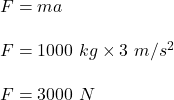## WILL MARK BRAINLLEST What is the net force on a 1,000 Kg object accelerating at 3 m/s2 this last one is a exponent use this formula net

Question

WILL MARK BRAINLLEST
What is the net force on a 1,000 Kg object accelerating at 3 m/s2 this last one is a exponent use this formula net force = mass x accelerat

in progress 0
2 weeks 2021-09-05T15:48:16+00:00 1 Answers 0 views 0

Net force is 3000 N

Explanation:

We have,

Mass of the object is 1000 kg

Acceleration of the object is 3 m/s²

It is required to find the net force acting on the object. The net force acting on the object is given by :So, the net force of 3000 N is acting on the object.